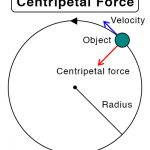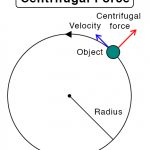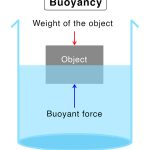Home / Physics / Balanced Force

# Balanced Force

A force is a physical entity that is applied to an object in order to displace it. The ultimate effect is to push or pull the object. A force is a vector that has both magnitude and direction.

## What is Balanced Force

Balanced forces refer to a set of forces acting on an object such that they do not affect its motion. Two equal and opposite forces will nullify each other. The net force on the object is zero.

What happens when forces are balanced?

Below are some facts about the balanced force. The effect that balanced forces have on an object are as follows:

• Does not cause a change in the motion of an object, which means a stationary object remains motionless, and a moving object continues to move in the same direction at a constant velocity
• The sum of all forces acting on the object along a line is always equal to zero

## Examples of Balanced Force

• An object suspended in water without any support
• A game of tug-of-war where neither team moves
• A car moving with a constant speed using cruise control
• Two players in an arm-wrestling game applying the same force
• A book lying on a table

## Balanced Force Equation

Suppose F1 and F2 are the magnitudes of two forces acting on an object in opposite directions. Then, by definition of balanced force, the net force acting on the object is zero.

Balanced Force Formula: F1 – F2 = 0

For example, a book of mass m is lying on a table with its weight mg, where g is the acceleration due to gravity. According to Newton’s law, the table will exert an equal and opposite force, known as the normal force FN. The two forces will cancel each other. The object will remain stationary along the line of action of the two forces.

Balanced Force Equation: FN – mg = 0

## Balanced vs. Unbalanced Forces

Differences

Unlike the balanced force, which does not change the motion of an object, unbalanced forces refer to a set of forces that do not nullify each other and result in a net (resultant) force. The effect of unbalanced forces is to displace a stationary object or change the direction of a moving object. In both cases, the object moves with an acceleration in the resultant force’s direction. It continues to move as long as this force exists.

Similarities

Like unbalanced forces move objects, an object can move even if balanced forces exist. For example, a force is applied to an object such that it moves against friction with a constant velocity. Therefore, it does not have acceleration, and the net force is zero.

## FAQ

Q.1. What forces balance gravity?

Ans. The buoyant force balances gravity.

Q.2. What forces balance in a main-sequence star?

Ans. The gravitational force balances the main sequence stars.

Article was last reviewed on Thursday, December 31, 2020

### Related articlesCentripetal ForceCentrifugal ForceBuoyancy